# Angles

Here you will learn about angles, including parts of angles, measuring angles, types of angles, and special pairs of angles.

Students first learn about angles in 4th grade with their work in geometric measurements. They expand that knowledge as they progress through middle school.

## What are angles?

Angles are formed where two rays or lines meet at a common point. The common point is called the vertex of the angle and the rays are called the arms of the angle.

The word angle comes from the Latin word “angulus” meaning corner.

Angles can be named by the points that exist on the arms and vertex.

For example,

This angle is named ‘ABC’ with the vertex letter in the middle.

### Types of angles

\hspace{2.8cm} Different Types of Angles

Type of AngleDescriptionDiagram
Acute angleAn angle whose measure
is less than 90^{\circ} but
greater than 0^{\circ}
Right angleAn angle that measure
exactly 90^{\circ}. Right angles
will have a square like
symbol showing that they
are equal to 90^{\circ}
Obtuse angleAn angle whose measure
is greater than 90^{\circ}
but less than 180^{\circ}
Straight angleAn angle whose measure
is equal to 180^{\circ}
Reflex angleAn angle whose measure
is greater than 180^{\circ}
and less than 360^{\circ}

Step-by-step guide: Types of angles

Step-by-step guide: Acute angles

Step-by-step guide: Obtuse angles

Step-by-step guide: Right angles

### Measuring angles

Angles are measured in degrees and have the degree sign ^{\circ}. The tool used for measuring angles is called a protractor.

The degree measure on the top center of a protractor is 90^{\circ}. Notice that the numbers left and right of the center either go up by ten or go down by ten.

If the angle is acute, you will use the acute measurement, and if the angle is obtuse, you will use the obtuse measurement.

For example, let’s look at the measure of ∠ A.

The vertex, A, of the angle is placed on the bottom center of the protractor. One arm of the angle is lined up with the bottom of the protractor at 0^{\circ}. The other arm is used to measure the turn from one arm to the next.

Notice how the arm goes through the measure of 60^{\circ} and 120^{\circ}. Since the angle is obtuse, use the measurement that is greater than 90^{\circ} and less than 180^{\circ}. The angle measure 120^{\circ}.

Step-by-step guide: Measuring angles

### Special pairs of angles

Angle rules are facts that we can apply to calculate missing angles in a diagram.

The five key angle facts that are used widely within the topic are:

Angle RuleDescriptionDiagram
arm and a vertex.

Angle CBD and Angle
Complementary anglesA pair of angles that
have a sum of 90^{\circ}.

These angles can be

If any two angles sum to
90^{\circ} they are
complementary.
Supplementary anglesThe sum of supplementary
angles is 180^{\circ}.

These angles can be

If any two angles sum to
180^{\circ} they are
supplementary.
Vertical anglesAngles that are equal in
measurement and formed
by intersecting lines.

Step-by-step guide: Complementary angles

Step-by-step guide: Supplementary angles

### What are angles?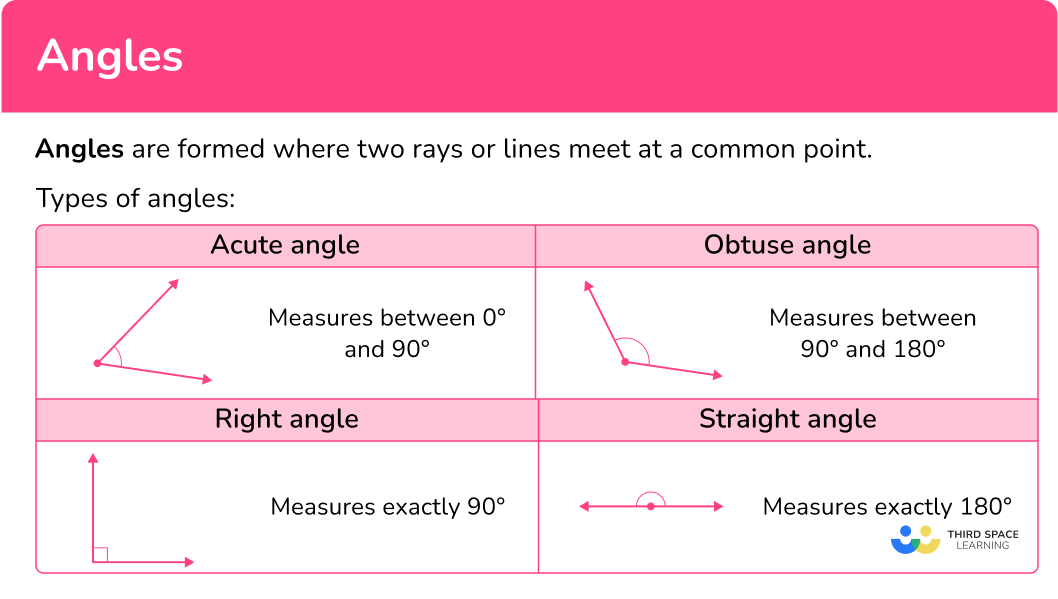## Common Core State Standards

How does this apply to 4th grade math and 7th grade math?

• Grade 4 – Measurement and Data (4.MD.C.5.a)
An angle is measured with reference to a circle with its center at the common endpoint of the rays, by considering the fraction of the circular arc between the points where the two rays intersect the circle. An angle that turns through 1/360 of a circle is called a “one-degree angle,” and can be used to measure angles.

• Grade 4 – Measurement and Data (4.MD.C.5.b)
An angle that turns through n one-degree angles is said to have an angle measure of n degrees.

• Grade 4 – Measurement and Data (4.MD.C.6)
Measure angles in whole-number degrees using a protractor. Sketch angles of specified measure.

• Grade 4 – Measurement and Data (4.MD.C.7)
Recognize angle measure as additive. When an angle is decomposed into non-overlapping parts, the angle measure of the whole is the sum of the angle measures of the parts. Solve addition and subtraction problems to find unknown angles on a diagram in real world and mathematical problems, for example, by using an equation with a symbol for the unknown angle measure.

• Grade 4 – Geometry (4.G.A.1)
Draw points, lines, line segments, rays, angles (right, acute, obtuse), and perpendicular and parallel lines. Identify these in two-dimensional figures.

• Grade 7 – Geometry (7.G.B.5)
Use facts about supplementary, complementary, vertical, and adjacent angles in a multi-step problem to write and solve simple equations for an unknown angle in a figure.

## How to use angles

In order to identify parts of an angle:

1. Name the vertex.
2. Name the arms as rays.

There are a lot of ways to use angles. For more specific step-by-step guides, check out the individual pages linked in the “What are angles?” section above or read through the examples below.

## Angle examples

### Example 1: identifying parts of an angle

Name the arms and vertex of the angle.

1. Name the vertex.

Point M is the vertex because it’s the point where the two rays meet.

2Name the arms as rays.

Ray ML is one arm and the other arm is ray MN.

### Example 2: measuring angles

What is the measure of the angle?

Determine the type of angle.

Check to make sure the vertex is at the center of the protractor and one arm is lined up with the bottom of the protractor.

Find the degree measure.

### Example 3: classifying angles

Classify ∠ OMN as either acute, obtuse, right or straight.

Recall the definitions of the types of angles needed.

Explain how the angle fits the definition.

### Example 4: determining special pairs of angles

Classify the ∠ ABC and ∠ CBD as supplementary angles or complementary angles.

Recall the definitions of the special pairs angles needed.

Explain how the angle pair fits the definition.

### Example 5: finding missing angle measures

The pair of angles are supplementary. Find the measure of Angle B.

Recall the definition of the special pairs of angles.

Find the missing angle.

### Example 6: finding missing angle measures

The pair of angles are complementary. Find the measure of angle QRT.

Recall the definition of the special pairs of angles.

Find the missing angle.

### Teaching tips for angles

• Give students hand held protractors so that they can learn how to use a protractor to measure angles.

• Have students draw different types of angles using colored pencils and then measure them so that they can practice how to measure angles while also being creative.

• Instead of giving students practice worksheets, have them sketch and measure the different angle pairs instead to help them formulate their own understanding.

### Easy mistakes to make

• Measuring angles with a protractor when the diagrams are not drawn accurately
When the question states that the diagram is not drawn accurately, we cannot simply measure the missing angles using a protractor. We need to use angle facts to calculate the missing angle.

• Confusing the definitions of the types of angles
For example, thinking that an obtuse angle is one whose measure is between 0^{\circ} and 90^{\circ} instead of between 90^{\circ} and 180^{\circ}.

• Confusing the sum of complementary and supplementary angles
For example, thinking that complementary angles sum to 180^{\circ} and that supplementary angles sum to 90^{\circ} when, in fact, complementary angles have a sum of 90^{\circ} and supplementary angles have a sum of 180^{\circ}.

### Practice angles questions

1. What is the vertex of the angle?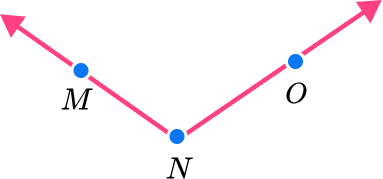OOMNMThe vertex of an angle is where the two rays meet, or where the two arms meet.

In this case, point N is where the two rays meet.

2. Choose the correct classification of the ∠ A.acute angleobtuse anglestraight anglereflex angleThe definition of an acute angle is an angle whose measure is between 0^{\circ} and 90^{\circ}.

∠ A is less than 90^{\circ} , so it is an acute angle.

3. Choose the correct classification of the angle.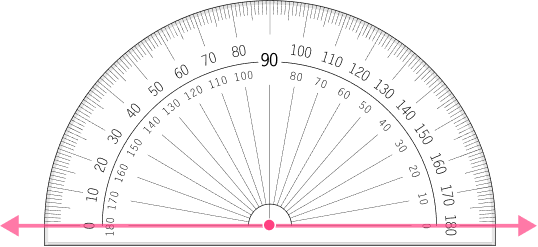acute angleright anglestraight anglereflex angleThe definition of a straight angle is an angle that measures 180^{\circ}.

On the protractor, you can see that the angle measures 180^{\circ} , so it is a straight angle.

4. Choose the correct classification of the angle.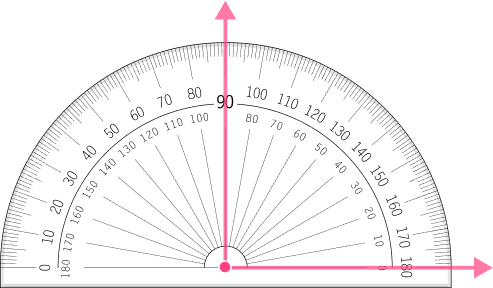acute angleobtuse anglestraight angleright angleThe definition of a right angle is an angle that measures 90^{\circ}.

On the protractor, you can see that the angle measures 90^{\circ} , so it is at a right angle.

5. ∠ A and ∠ B are complementary angles. Find the measure of ∠ B.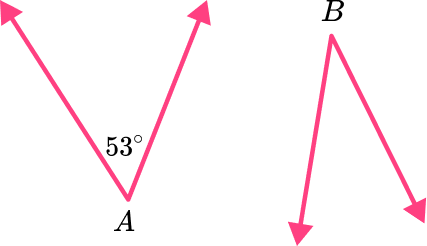37^{\circ}47^{\circ}127^{\circ}137^{\circ}Complementary angles are a pair of angles whose sum is 90^{\circ}.

∠ A is 53^{\circ} , so to find ∠ B subtract 53 from 90.

90-53=37

∠ B is 37^{\circ} .

6. ∠ J and ∠ K are supplementary angles. Find ∠ J.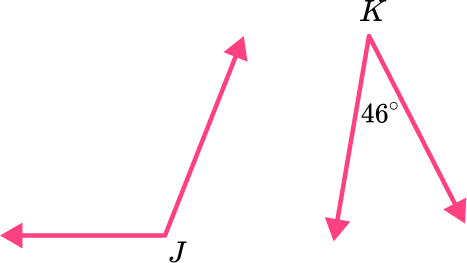46^{\circ}44^{\circ}124^{\circ}134^{\circ}Supplementary angles are a pair of angles whose sum is 180^{\circ}.

∠ K is 46^{\circ} , so to find ∠ J subtract 46 from 180.

180-46=134

∠ J is 134^{\circ} .

## Angle FAQs

Are there only positive angle measurements?

The angle measurements you will be working with in elementary school and middle school are positive angle measurements. When you progress into high school you will work with negative angle measurements.

How are types of angles used in classification of polygons?

Understanding the types of angles will help you understand the angles of a triangle. Triangles are classified by their angles and their sides.

Are degrees the only unit of measurement for angles?

No, radians can be used as a unit of measurement for angles.  However, you will not use radian measurements until high school.

What are alternate interior angles?

Special angle pairs are formed from parallel lines and a transversal line intersecting the parallel lines. One of those special pair of angles formed is called alternate interior angles. There are other angles that are formed too such as alternate exterior angles and corresponding angles.

Is a straight line a straight angle?

A straight angle always forms a straight line.

## Still stuck?

At Third Space Learning, we specialize in helping teachers and school leaders to provide personalized math support for more of their students through high-quality, online one-on-one math tutoring delivered by subject experts.

Each week, our tutors support thousands of students who are at risk of not meeting their grade-level expectations, and help accelerate their progress and boost their confidence.